$$\newcommand{\id}{\mathrm{id}}$$ $$\newcommand{\Span}{\mathrm{span}}$$ $$\newcommand{\kernel}{\mathrm{null}\,}$$ $$\newcommand{\range}{\mathrm{range}\,}$$ $$\newcommand{\RealPart}{\mathrm{Re}}$$ $$\newcommand{\ImaginaryPart}{\mathrm{Im}}$$ $$\newcommand{\Argument}{\mathrm{Arg}}$$ $$\newcommand{\norm}{\| #1 \|}$$ $$\newcommand{\inner}{\langle #1, #2 \rangle}$$ $$\newcommand{\Span}{\mathrm{span}}$$

# 4.E: The Definite Integral (Exercises)

$$\newcommand{\vecs}{\overset { \rightharpoonup} {\mathbf{#1}} }$$ $$\newcommand{\vecd}{\overset{-\!-\!\rightharpoonup}{\vphantom{a}\smash {#1}}}$$$$\newcommand{\id}{\mathrm{id}}$$ $$\newcommand{\Span}{\mathrm{span}}$$ $$\newcommand{\kernel}{\mathrm{null}\,}$$ $$\newcommand{\range}{\mathrm{range}\,}$$ $$\newcommand{\RealPart}{\mathrm{Re}}$$ $$\newcommand{\ImaginaryPart}{\mathrm{Im}}$$ $$\newcommand{\Argument}{\mathrm{Arg}}$$ $$\newcommand{\norm}{\| #1 \|}$$ $$\newcommand{\inner}{\langle #1, #2 \rangle}$$ $$\newcommand{\Span}{\mathrm{span}}$$ $$\newcommand{\id}{\mathrm{id}}$$ $$\newcommand{\Span}{\mathrm{span}}$$ $$\newcommand{\kernel}{\mathrm{null}\,}$$ $$\newcommand{\range}{\mathrm{range}\,}$$ $$\newcommand{\RealPart}{\mathrm{Re}}$$ $$\newcommand{\ImaginaryPart}{\mathrm{Im}}$$ $$\newcommand{\Argument}{\mathrm{Arg}}$$ $$\newcommand{\norm}{\| #1 \|}$$ $$\newcommand{\inner}{\langle #1, #2 \rangle}$$ $$\newcommand{\Span}{\mathrm{span}}$$

## 4.1: Determining Distance Traveled from Velocity

1. Along the eastern shore of Lake Michigan from Lake Macatawa (near Holland) to Grand Haven, there is a bike bath that runs almost directly north-south. For the purposes of this problem, assume the road is completely straight, and that the function s(t) tracks the position of the biker along this path in miles north of Pigeon Lake, which lies roughly halfway between the ends of the bike path. Suppose that the biker’s velocity function is given by the graph in Figure 4.9 on the time interval 0 ≤ t ≤ 4 (where t is measured in hours), and that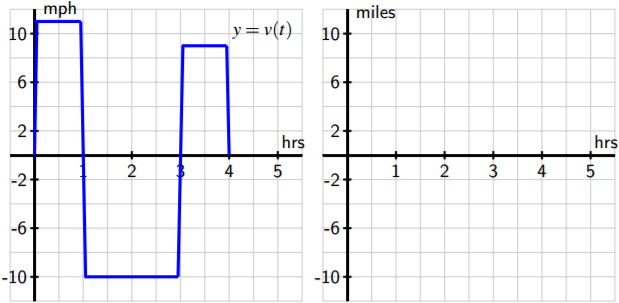Figure 4.9: The graph of the biker’s velocity, y = v(t), at left. At right, axes to plot an approximate sketch of y = s(t).

(a) Approximately how far north of Pigeon Lake was the cyclist when she was the greatest distance away from Pigeon Lake? At what time did this occur?

(b) What is the cyclist’s total change in position on the time interval 0 ≤ t ≤ 2? At t = 2, was she north or south of Pigeon Lake?

(c) What is the total distance the biker traveled on 0 ≤ t ≤ 4? At the end of the ride, how close was she to the point at which she started?

(d) Sketch an approximate graph of y = s(t), the position function of the cyclist, on the interval 0 ≤ t ≤ 4. Label at least four important points on the graph of s.

2. A toy rocket is launched vertically from the ground on a day with no wind. The rocket’s vertical velocity at time t (in seconds) is given by v(t) = 500 − 32t feet/sec.

(a) At what time after the rocket is launched does the rocket’s velocity equal zero? Call this time value a. What happens to the rocket at t = a?

(b) Find the value of the total area enclosed by y = v(t) and the t-axis on the interval 0 ≤ t ≤ a. What does this area represent in terms of the physical 219 setting of the problem?

(c) Find an antiderivative s of the function v. That is, find a function s such that s 0 (t) = v(t).

(d) Compute the value of s(a) − s(0). What does this number represent in terms of the physical setting of the problem?

(e) Compute s(5) − s(1). What does this number tell you about the rocket’s flight?

3. An object moving along a horizontal axis has its instantaneous velocity at time t in seconds given by the function v pictured in Figure 4.10, where v is measured in feet/sec. Assume that the curves that make up the parts of the graph of y = v(t) are eitherFigure 4.10: The graph of y = v(t), the velocity function of a moving object.

portions of straight lines or portions of circles.

(a) Determine the exact total distance the object traveled on 0 ≤ t ≤ 2.

(b) What is the value and meaning of s(5) − s(2), where y = s(t) is the position function of the moving object?

(c) On which time interval did the object travel the greatest distance: [0, 2], [2, 4], or [5, 7]?

(d) On which time interval(s) is the position function s increasing? At which point(s) does s achieve a relative maximum?

4. Filters at a water treatment plant become dirtier over time and thus become less effective; they are replaced every 30 days. During one 30-day period, the rate at which pollution passes through the filters into a nearby lake (in units of particulate matter per day) is measured every 6 days and is given in the following table. The time t is measured in days since the filters were replaced.(a) Plot the given data on a set of axes with time on the horizontal axis and the rate of pollution on the vertical axis.

(b) Explain why the amount of pollution that entered the lake during this 30-day period would be given exactly by the area bounded by y = p(t) and the t-axis on the time interval [0, 30].

(c) Estimate the total amount of pollution entering the lake during this 30-day period. Carefully explain how you determined your estimate.

## 4.2: Riemann Sums

1. Consider the function f (x) = 3x + 4.

(a) Compute M4 for y = f (x) on the interval [2, 5]. Be sure to clearly identify the value of 4x, as well as the locations of x0, x1, . . ., x4. Include a careful sketch of the function and the corresponding rectangles being used in the sum.

(b) Use a familiar geometric formula to determine the exact value of the area of the region bounded by y = f (x) and the x-axis on [2, 5].

(c) Explain why the values you computed in (a) and (b) turn out to be the same. Will this be true if we use a number different than n = 4 and compute Mn? Will L4 or R4 have the same value as the exact area of the region found in (b)?

(d) Describe the collection of functions g for which it will always be the case that Mn, regardless of the value of n, gives the exact net signed area bounded between the function g and the x-axis on the interval [a, b].

2. Let S be the sum given by S = ((1.4) 2+1)·0.4+((1.8) 2+1)·0.4+((2.2) 2+1)·0.4+((2.6) 2+1)·0.4+((3.0) 2+1)·0.4.

(a) Assume that S is a right Riemann sum. For what function f and what interval [a, b] is S an approximation of the area under f and above the x-axis on [a, b]? Why? 232 4.2. RIEMANN SUMS

(b) How does your answer to (a) change if S is a left Riemann sum? a middle Riemann sum?

(c) Suppose that S really is a right Riemann sum. What is geometric quantity does S approximate?

(d) Use sigma notation to write a new sum R that is the right Riemann sum for the same function, but that uses twice as many subintervals as S.

3. A car traveling along a straight road is braking and its velocity is measured at several different points in time, as given in the following table.(a) Plot the given data on a set of axes with time on the horizontal axis and the velocity on the vertical axis.

(b) Estimate the total distance traveled during the car the time brakes using a middle Riemann sum with 3 subintervals.

(c) Estimate the total distance traveled on [0, 1.8] by computing L6, R6, and 1 2 (L6 + R6).

(d) Assuming that v(t) is always decreasing on [0, 1.8], what is the maximum possible distance the car traveled before it stopped? Why?

4. The rate at which pollution escapes a scrubbing process at a manufacturing plant increases over time as filters and other technologies become less effective. For this particular example, assume that the rate of pollution (in tons per week) is given by the function r that is pictured in Figure 4.18.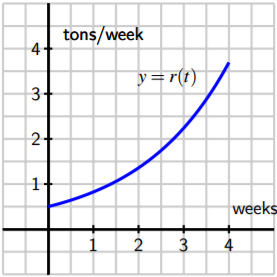Figure 4.18: The rate, r(t), of pollution in tons per week.

(a) Use the graph to estimate the value of M4 on the interval [0, 4]. 4.2. RIEMANN SUMS 233

(b) What is the meaning of M4 in terms of the pollution discharged by the plant?

(c) Suppose that r(t) = 0.5e 0.5t . Use this formula for r to compute L5 on [0, 4].

(d) Determine an upper bound on the total amount of pollution that can escape the plant during the pictured four week time period that is accurate within an error of at most one ton of pollution.

## 4.3: The Definite Integral

1. The velocity of an object moving along an axis is given by the piecewise linear function v that is pictured in Figure 4.29. Assume that the object is moving to the right when its 4.3. THE DEFINITE INTEGRAL 247 velocity is positive, and moving to the left when its velocity is negative. Assume that the given velocity function is valid for t = 0 to t = 4.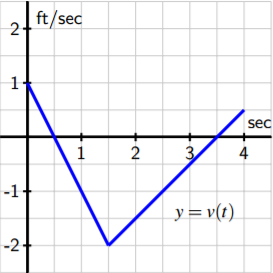Figure 4.29: The velocity function of a moving object.

(a) Write an expression involving definite integrals whose value is the total change in position of the object on the interval [0, 4].

(b) Use the provided graph of v to determine the value of the total change in position on [0, 4].

(c) Write an expression involving definite integrals whose value is the total distance traveled by the object on [0, 4]. What is the exact value of the total distance traveled on [0, 4]?

(d) What is the object’s exact average velocity on [0, 4]?

(e) Find an algebraic formula for the object’s position function on [0, 1.5] that satisfies s(0) = 0.

2. Suppose that the velocity of a moving object is given by v(t) = t(t − 1)(t − 3), measured in feet per second, and that this function is valid for 0 ≤ t ≤ 4.

(a) Write an expression involving definite integrals whose value is the total change in position of the object on the interval [0, 4].

(b) Use appropriate technology (such as http://gvsu.edu/s/a99 ) to compute Riemann sums to estimate the object’s total change in position on [0, 4]. Work to ensure that your estimate is accurate to two decimal places, and explain how you know this to be the case.

(c) Write an expression involving definite integrals whose value is the total distance traveled by the object on [0, 4]. 9Marc Renault, Shippensburg University. 248 4.3. THE DEFINITE INTEGRAL

(d) Use appropriate technology to compute Riemann sums to estimate the object’s total distance travelled on [0, 4]. Work to ensure that your estimate is accurate to two decimal places, and explain how you know this to be the case.

(e) What is the object’s average velocity on [0, 4], accurate to two decimal places? 3. Consider the graphs of two functions f and g that are provided in Figure 4.30. Each piece of f and g is either part of a straight line or part of a circle.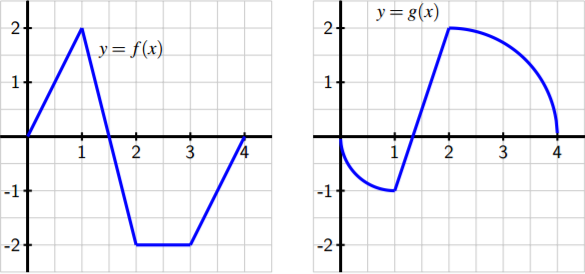Figure 4.30: Two functions f and g.

(a) Determine the exact value of R 1 0 [ f (x) + g(x)] dx.

(b) Determine the exact value of R 4 1 [2 f (x) − 3g(x)] dx.

(c) Find the exact average value of h(x) = g(x) − f (x) on [0, 4].

(d) For what constant c does the following equation hold? Z 4 0 c dx = Z 4 0 [ f (x) + g(x)] dx

4. Let f (x) = 3 − x 2 and g(x) = 2x 2 .

(a) On the interval [−1, 1], sketch a labeled graph of y = f (x) and write a definite integral whose value is the exact area bounded by y = f (x) on [−1, 1].

(b) On the interval [−1, 1], sketch a labeled graph of y = g(x) and write a definite integral whose value is the exact area bounded by y = g(x) on [−1, 1].

(c) Write an expression involving a difference of definite integrals whose value is the exact area that lies between y = f (x) and y = g(x) on [−1, 1].

(d) Explain why your expression in (c) has the same value as the single integral R 1 −1 [ f (x) − g(x)] dx.

(e) Explain why, in general, if p(x) ≥ q(x) for all x in [a, b], the exact area between y = p(x) and y = q(x) is given by Z b a [p(x) − q(x)] dx.

## Exercises

1. The instantaneous velocity (in meters per minute) of a moving object is given by the function v as pictured in Figure 4.37. Assume that on the interval 0 ≤ t ≤ 4, v(t) is given by v(t) = − 1 4 t 3 + 3 2 t 2 + 1, and that on every other interval v is piecewise linear, as shown.

(a) Determine the exact distance traveled by the object on the time interval 0 ≤ t ≤ 4.

(b) What is the object’s average velocity on [12, 24]?

(c) At what time is the object’s acceleration greatest?

(d) Suppose that the velocity of the object is increased by a constant value c for all values of t. What value of c will make the object’s total distance traveled on [12, 24] be 210 meters?Figure 4.37: The velocity function of a moving body.

2. A function f is given piecewise by the formula f (x) =     −x 2 + 2x + 1, if 0 ≤ x < 2 −x + 3, if 2 ≤ x < 3 x 2 − 8x + 15, if 3 ≤ x ≤ 5

(a) Determine the exact value of the net signed area enclosed by f and the x-axis on the interval [2, 5].

(b) Compute the exact average value of f on [0, 5].

(c) Find a formula for a function g on 5 ≤ x ≤ 7 so that if we extend the above definition of f so that f (x) = g(x) if 5 ≤ x ≤ 7, it follows that R 7 0 f (x) dx = 0.

3. When an aircraft attempts to climb as rapidly as possible, its climb rate (in feet per minute) decreases as altitude increases, because the air is less dense at higher altitudes. Given below is a table showing performance data for a certain single engine aircraft, giving its climb rate at various altitudes, where c(h) denotes the climb rate of the airplane at an altitude h.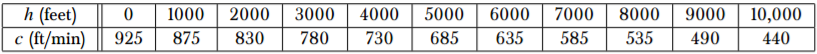Let a new function called m(h) measure the number of minutes required for a plane at altitude h to climb the next foot of altitude.

(a) Determine a similar table of values for m(h) and explain how it is related to the table above. Be sure to explain the units.

(b) Give a careful interpretation of a function whose derivative is m(h). Describe what the input is and what the output is. Also, explain in plain English what the function tells us. 264 4.4. THE FUNDAMENTAL THEOREM OF CALCULUS

(c) Determine a definite integral whose value tells us exactly the number of minutes required for the airplane to ascend to 10,000 feet of altitude. Clearly explain why the value of this integral has the required meaning.

(d) Use the Riemann sum M5 to estimate the value of the integral you found in (c). Include units on your result.

4. In Chapter 1, we showed that for an object moving along a straight line with position function s(t), the object’s “average velocity on the interval [a, b]” is given by AV[a,b] = s(b) − s(a) b − a . More recently in Chapter 4, we found that for an object moving along a straight line with velocity function v(t), the object’s “average value of its velocity function on [a, b]” is vAVG[a,b] = 1 b − a Z b a v(t) dt. Are the “average velocity on the interval [a, b]” and the “average value of the velocity function on [a, b]” the same thing? Why or why not? Explain.

4.E: The Definite Integral (Exercises) is shared under a CC BY-SA 4.0 license and was authored, remixed, and/or curated by Matthew Boelkins, David Austin & Steven Schlicker (ScholarWorks @Grand Valley State University) via source content that was edited to conform to the style and standards of the LibreTexts platform; a detailed edit history is available upon request.